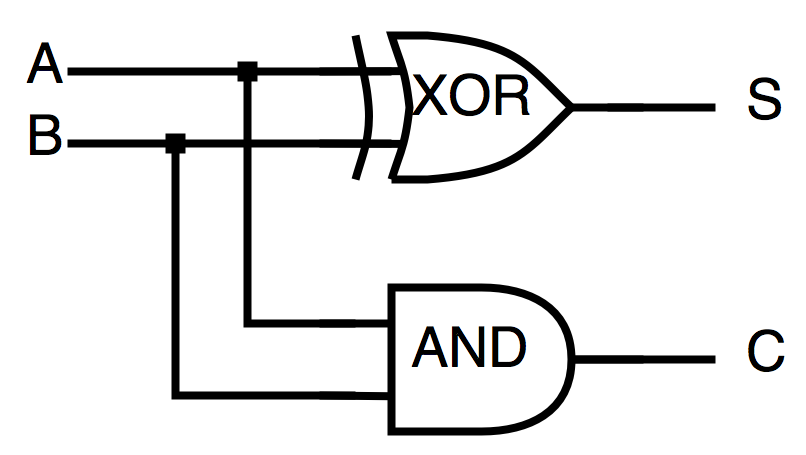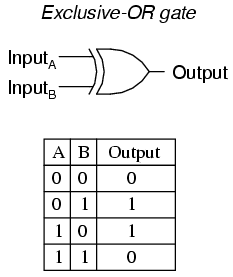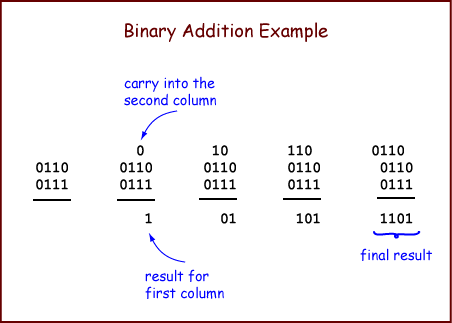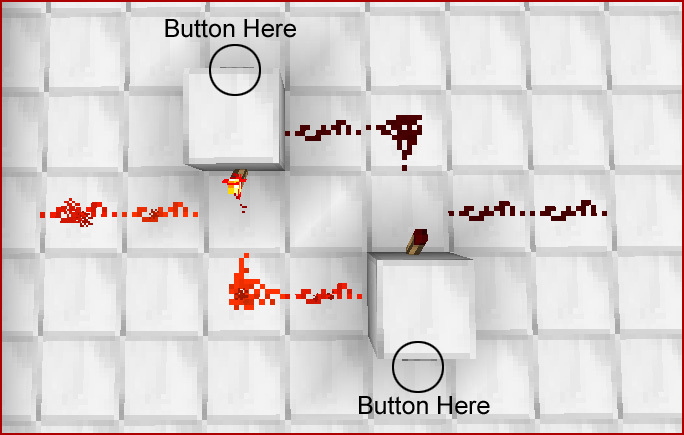This is the blog of Will Badart.

# Origins of Computers

14 Jan 2019

• #computers
• #hardware

This installment of How We Tricked Rocks Into Thinking will glide over the history of computing machines and introduce you to the fundamental building blocks of digital systems.

## Genesis

Computers in general are much older than the modern digital devices with which we’re all familiar. The first general purpose computers were of course people; people responsible for computing things. Some sources date the term “computer” back to the 17th century.

Computing machines have also been with us for some time. The abacus, for instance, may have been invented by our species’ first civilization, the Sumerians, some 5,000 years ago.A Chinese abacus (source)

Fast forward to the 1800s, and we find that numerical tables supporting navigation and engineering endeavors are still computed by hand, and therefore subject to human error.

This was the motivation for Charles Babbage’s research into mechanical computers. Babbage designed (and started to construct) the Difference Engine: a machine to compute polynomials. Building on this work, he later designed the Analytical Engine: history’s first glance at a programmable digital computer. Ada Lovelace is credited with designing an algorithm which would have run on the engine, becoming the world’s first programmer before the invention of its first programming language.

## Going Digital

How could such a machine have worked? Just like us humans, the machine needs representations of values, and mechanics for combining them in the desired fashion. We have axons and dendrites and such to help us with that, but early digital computers were much simpler.

Imagine a light switch that controls another switch, rather than a lightbulb, and, depending on the wiring, will have slightly different behavior. These switches control each other by sending one of two messages: “yes” or “no.”

One of the possible behaviors is: if the first switch is on (sending a “yes”), then the second one always emits a “yes” (even if its switch is down). This configuration is called an OR gate since the signal emitted by the circuit is a “yes” if either the first OR the second switch is up.

Another possibility is to wire the two switches such that if the first switch is down, the second switch never emits a yes, even if its switch is up. This is the AND gate since, in order for the circuit to emit a yes, both the first AND second switches must be up.Truth tables and logic gate diagrams for AND and OR (source)

The top of this diagram depicts the two “logic gates” we just learned. The bottom half shows the “truth table” of each gate. Truth tables enumerate the outputs for all possible inputs of a logical proposition. To read it, simply say “0 AND 0 is 0,” or “1 OR 0 is 1” etc. In our lightbulb metaphor, 0 is a “no” message and “1” is a “yes.”

This is what it means to be a binary, digital system. Computers fundamentally only manipulate two (hence binary) discrete (hence digital) values of “yes” and “no.” Any higher abstractions like numbers, words, or programs, are just that: abstractions, composed from different streams of yesses and noes (I had to look up the plural forms). Note that I use the metaphor of electrical light switches, but any hardware capable of manipulating yesses and noes can be a digital computer. You can even make a computer out of dominoes.

## Thinking Digital

Let’s look into how we represent numbers on computers. But, before we start thinking about circuits and switches, a brief analog exercise:

Each finger individually only carries one bit of information (am I up, or am I or folded down?), but with a simple logical system for combining each of those ten bits, we can represent a thousand numbers. (NOTE: If you’re still feeling shaky on the concept of counting in base 2, I’d encourage you to watch the video again! This is important.)

Computers don’t have fingers, but wires, each of which either carries a current or doesn’t. In modern systems, we use not ten but 64 bits to represent numbers. We used to use 32. Details on that.

One component that uses numbers is the adder, which (you guessed it) takes in two numbers and spits out their sum. u/MysteriousHobo2, to whom I also credit the above light switch metaphor, put it better than I could (light formatting added):

We want to add just like normal adding. Let’s look at just the rightmost digit - it can be either `0` (no) or `1` (yes), and we’re trying to add it to another digit that’s either `0` or `1`. At first, we might try something like an OR gate. Then `0 + 0 = 0`, `0 + 1 = 1`, and `1 + 0 = 1`, which looks good so far. Except that `1 + 1` will also give us `1`, which we don’t want.

Remember, `1 + 1` is still `2`, but we need to arrive at the representation of `2` in binary; we need another digit in order to form the binary `10`.

We want to get `0` and carry a `1` to the left (remember, we can’t create the digit 2, we have to represent 2 as “`10`”). So what we actually want is something called a XOR, that’s a fancy name for “[OR and not AND]”. We take the result of an OR gate, and we AND it with the flipped result of an AND gate. So we’ll have `0 + 0 = 0`, `0 + 1 = 1`, `1 + 0 = 1`, and `1 + 1 = 0`. To make sure we’re actually adding 1 and 1, and not just erasing it to 0, we also need to record a carry digit, but that’s just an AND gate: if both the first AND second thing are 1, carry a 1, otherwise carry a 0.Visual representation of the adding process. 'S' is the sum bit and 'C' is the carry-over bit. (source)

[Next], we do the same thing one step to the left, but we also include the carry digit if we have one. We XOR the digits to see if we should record a 1 or a 0 in this position, and if we have 2 or more 1s (in gates we know, one way to write that is “‘a and b’ or ‘b and c’ or ‘a and c’”) we carry a 1 to the next position.

Here’s a diagram and truth table of the XOR gate, to try and elucidate its mechanics a little better:XOR gate symbol and truth table (source)

And here’s a numerical example of the above adding process in action:Example of binary addition in written, rather than circuit form (source)

Try to find the different gates mentioned hiding in the numerical example. Observe how XOR influences the bit that’s written below the line and how AND influences the carried bit above. I encourage you to refer back to the truth tables heavily.

Unless you’re using this post to study for a logic design test, you don’t need to understand every bit and wire beneath this process. Simply take away that we can build incrementally more abstract (and powerful) combinations of yesses and noes which represent incrementally more rich information.

## Started From the Bottom

It’s time to shed the light switch metaphor and discover the actual medium that carries our yesses and noes. We’re talking about the transistor .

A transistor is a tiny electrical component with three wires: input, output, and control. The control wire determines whether current from the input can flow to the output. When we talk about the logical inputs to our gates, we’re talking about the signals carried on the control wires. For example, check out this circuit diagram:A transistor-level diagram (source)

Given that A and B are the controls, and current flows from the input at the top, downwards to the output (and ground) at the very bottom, can you guess which logic gate it represents?

Let’s walk though it. Starting from the input at the top and working down, we first hit transistor A. If A is “yes,” the current continues, otherwise it stops. This means that “A is no” implies “Out is no.” Assuming A is “yes,” the current will reach transistor B. If B is “yes,” the current continues to “Out,” otherwise it does not. This means that “A is yes and B is yes implies Out is yes,” while “A is yes and B is no implies Out is no.”

Check the truth tables above, and you’ll see that this pattern corresponds to AND. Different wirings give rise to different gates. As an exercise, which wires would you have to draw (or remove) to convert the above diagram into an OR gate? Hint: follow the truth table.

### Memory

In order to do anything truly interesting, we need to be able to design circuit components capable of remembering a bit. For brevity’s sake, I won’t describe their guts in detail, but if you’re curious, look into latches and then flip flops. I only mean to make you aware of their existence so you don’t have to suspend your disbelief when I later mention memory in circuits.

I actually found a great post about these components on the Minecraft wiki. The game includes mechanics for building circuits from the primitives we’re discussing here.A latch circuit, implemented using Minecraft's redstone feature (source)

## Inputs and Outputs

Thus are the tiniest units of a computer. We now have a rough idea of how circuits can manipulate bits (and therefore numbers) in isolation, but we need more information to understand the computer as an interactive system. We need inputs and outputs, or I/O.

Hold in your mind the simple little calculators we used in elementary school. Basically nothing but a number pad and 4 arithmetic operators (forget about the memory buttons for now). We’re going to examine where the bits go when you press `4`, `2`, `/`, `7`, then `=`, aka `42 / 7 =`.

### Input

First we need to “create” our representation of `42`. When we first enter a `4`, all the circuit knows is that it sees a four, so it writes that down. When the `2` comes in, we realize that the `4` was meant to be in the tens place, that is, that it really represents a `40`, so we multiply it by 10 and only then add in the `2`.

This pattern of shifting and replacing would continue for any further digits entered (e.g. if we saw a `3`, we’d realize that `42` is actually `420`, so we’d multiply by 10 and add in the 3 to reach `423`), but instead we get a special input which signifies an operator. It’s up to the designer which input signals map to each operator, so it doesn’t matter to us which value pressing `/` sends to the circuit, only that it triggers the next stage of calculation, namely, writing down the first operand (`42`) and the operator (`/`).

We can now begin collecting the digits of the second operand in the same way we did the first. We stop collecting digits when we get the GO signal; when `=` is pressed.

This sends the two operands through a unique circuit, determined by the recorded operator, which outputs the result of the given calculation.

### Output

All the while, there has been a circuit sitting atop the “current” number (be it the `42` while we were entering it, or the `6` once we press enter) which translates it into input for the seven-segment display. Each digit of the display has seven segments, each of which is either on or off. The circuit encodes a translation from a digit (e.g. `3`, or `11` in binary) to a pattern of segments which, when on, represent the number (e.g. 3 is “all segments except the left 2 vertical ones [`f` and `e` in the diagram below]”).Control wires to a 7-segment display (this one is technically 8, counting the decimal point). The "common anodes" basically carry power. (source)

It takes a little imagination, but we can extrapolate this to a computer. Instead of 10 or so buttons, we have a full keyboard, but it’s still just an array of buttons, each of which sends a unique signal to a processor circuit. Further, rather than a simplistic seven-segment display, you have a screen of pixels, but you still have a circuit (which may be virtual, i.e. made of software) that translates between some input numbers and a pattern of pixels to activate, showing different symbols.

## Conclusion, For Now

In this segment, we built up our understanding of digital logic design, and how we can build rich, useful information and operations out of nothing but yesses, noes, and clever arrangements of transistors.

Soon, we’ll build on this knowledge, moving away from simple circuits and bit-at-a-time operations and examining both the larger components they compose in a computer, and the paradigms that inform the design of those components.

Recent posts: